﻿ 精确测定地震计传递函数文章快速检索
 地震地磁观测与研究2018, Vol. 39Issue (1): 107-116  DOI: 10.3969/j.issn.1003-3246.2018.01.0180

### 引用本文Wang Hongti, Cui Rensheng, Li Jiang, et al. Precise determination of transfer function for feedback seismometer[J]. Seismological and Geomagnetic Observation and Research, 2018, 39(1): 107-116. DOI: 10.3969/j.issn.1003-3246.2018.01.018.

### 文章历史

1. 中国北京 100036 中国地震局地震预测研究所;
2. 中国北京 102628 北京港震仪器设备有限公司

Precise determination of transfer function for feedback seismometer
Wang Hongti1,2, Cui Rensheng1, Li Jiang1,2, Ye Peng2
1. Institute of Earthquake Forecasting, China Earthquake Administration, Beijing Municipality 100036, China;
2. Beijing Gangzhen Instrument and Equipment Corporation, Ltd., Beijing Municipality 102628, China
Abstract: A precise determination method of transfer function for a feedback seismometer with step and sine signal calibration is given in this paper. Firstly its natural period and damping rate is measured by step calibration, so the low frequency part of the transfer function is gotten. Then the full frequency band of response of the seismometer is calculated from online current measured sine signal calibration data. The transfer function of the seismometer is from fitting of the full frequency band of response with a model in accordance with intrinsic structure of the transfer function of a feedback seismometer. This method is special suitable to verification of transfer function, on site measurement of transfer function to know the long time stability of a seismometer.
Key Words: transfer function    criteria function    step calibration    sine calibration    accurate determination
0 引言

1 测试原理与方法 1.1 反馈式地震计传递函数表述

 $H\left(s \right) = {H_0}\left(s \right){H_1}\left(s \right)$ (1)
 ${H_0}\left(s \right) = \frac{{{s^2}}}{{{s^2} + 2{D_0}{\omega _0}s + \omega _0^2}}$ (2)
 ${H_1}\left(s \right) = \frac{{{b_m}{s^m} + {b_{m - 1}}{s^{m - 1}} + \cdots + {b_1}s + {b_0}}}{{{a_n}{s^n} + {a_{n - 1}}{s^{n - 1}} + \cdots + {a_1}s + {a_0}}}$ (3)

1.2 地震计自振周期与阻尼系数确定

 $r\left(t \right) = \frac{K}{{{\omega _0}\sqrt {1 - D_0^2} }}{e^{ - {D_0}{\omega _0}t}}\sin \left({{\omega _0}\sqrt {1 - D_0^2} t} \right)$ (4)
 ${R_0}\left(s \right) = K{H_0}\left(s \right) = \frac{K}{{{s^2} + 2{\omega _0}{D_0}s + \omega _0^2}}$ (5)

1.3 地震计响应特性精确测定及H1(s)求取

 $w\left(t \right) = A\sin \left({\omega t + \varphi } \right)\;\;\;\;\;\;\;t \in \left[ {{t_0}, {t_1}} \right]$ (6)

（1）对每一个频率、每一个通道和测量电压的波形取出一段同步数据，使用正弦波拟合方法，得到同一段地震计3个分向和测流电压的振幅和相位。

（2）计算地震计输出各频点的3个方向的相位响应。电流流过标定线圈产生驱动力推定摆锤运动，力与加速度成正比，地震计输出与速度量成比例的电压。因此，地震计响应在指定频率点相位响应为

 ${\varphi _r}\left(f \right) = {\varphi _s}\left(f \right) - {\varphi _I}\left(f \right) + \frac{{\rm{ \mathsf{ π} }}}{2}$ (7)

（3）计算实际标定电流。用获得的测流电压除以测流电阻值即得到实际标定电流。

（4）计算地震计幅频特性。使用已有地震计输出3个分向各频率的振幅、标定灵敏度Sc和实测标定电流值，按式（8）计算地震计幅频特性。

 ${A_r}\left(f \right) = \frac{{2{\rm{ \mathsf{ π} }}f{V_f}}}{{{S_c}{I_f}}}$ (8)

（5）计算地震计响应函数R(f)。将式（8）计算的地震计幅频特性带入式（9），可得

 $R\left(f \right) = {A_r}\left(f \right)\cos {\varphi _r}\left(f \right) + j{A_r}\left(f \right)\sin {\varphi _r}\left(f \right)$ (9)

（6）计算所得地震计传递函数在各频点的实测值Hm(f )。

 ${H_m}\left(f \right) = R\left(f \right)/\left| {R\left({{f_{{\rm{norm}}}}} \right)} \right|$ (10)

（7）拟合确定H1(s)。采用最优化方法拟合求H1(s)，其目标函数为

 ${\rm{Criteria}}\left({B, A} \right) = \sum\limits_{i = 1}^n {\left| {{H_m}\left(i \right) - {H_0}\left({{s_i}} \right) - {H_1}{{\left({{s_i}} \right)}^2}} \right|W\left(i \right)}$ (11)

1.4 测试电路设计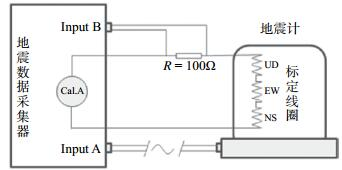图 1 精确测定地震计传递函数电路示意 Fig.1 The schematic diagram of measuring seismometer transfer function
2 测试实验及数据处理

2.1 实验仪器

2.2 阶跃标定确定周期和阻尼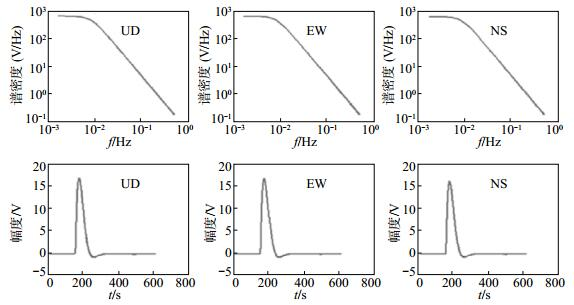图 2 地震计阶跃标定波形拟合结果 Fig.2 The waveform fitting result of step calibration of the seismometer表 1 阶跃标定拟合得到等效机械摆参数 Tab.1 The equivalent mechanical pendulum parameters from step calibration
2.3 正弦波标定获得地震计幅频特性和相频特性表 2 正弦波标定参数设计 Tab.2 Sine wave calibration parameters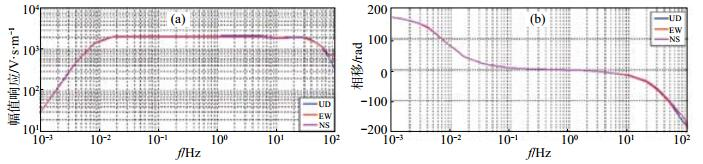图 3 实测标准地震计频率特性 (a)地震计幅频特性；(b)地震计相频特性 Fig.3 The actual measured frequency characteristics of standard seismometer表 3 实际测得的地震计幅频响应值 Tab.3 The actual measured amplitude frequency response of the seismometer表 4 实际测得的地震计相频响应值 Tab.4 The actual measured phase frequency response of the seismometer
2.4 拟合求地震计传递函数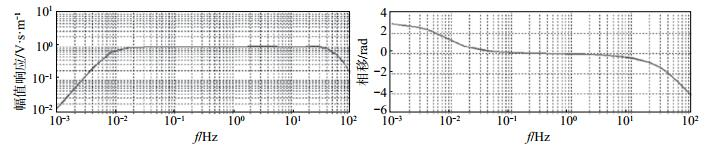图 4 幅频特性和相频特性传递函数拟合结果 Fig.4 The fitted frequency characteristics of the seismometer transfer function表 5 标准地震计UD向传递函数拟合结果 Tab.5 The fitted result of the UD component of the seismometer transfer function表 6 标准地震计EW向传递函数拟合结果 Tab.6 The fitted result of the EW component of the seismometer transfer function表 7 标准地震计NS向传递函数拟合结果 Tab.7 The fitted result of the NS component of the seismometer transfer function
3 结果分析

（1）地震计传递函数低频、高频部分的关系及各自作用。图 5清楚显示地震计传递函数可以分为低频段和高频段2个部分，其中低频段由其等效机械摆的周期和阻尼决定，高频段通过拟合求得。低频段和高频段综合在一起形成地震计的整体传递函数。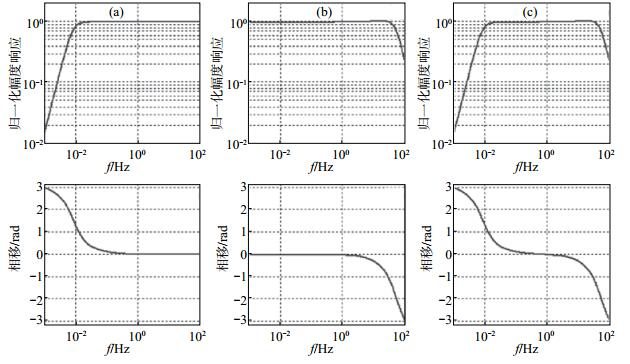图 5 拟合结果显示的低频段、高频段和综合频率特性 (a)低频段传递函数的幅频特性和相频特性；(b)传递函数高频段的幅频特性和相频特性；(c)综合幅频特性和相频特性 Fig.5 The fitted result of the low frequency band, high frequency band and full frequency band

（2）拟合结果的多解性及最终结果选取。根据选择的参数不同，同一频率特性可以有多个传递函数表达式，这些表达式在形式上是有差别的，是实际测量数据拟合得到的结果，只要与实际数据吻合，均可作为地震计传递函数。在实际工作中应当选用与实际数据拟合残差小于一定水平范围内最短数据长度的拟合结果。

（3）名义电流和实际电流误差对测试的影响。表 8给出本实验中名义电流与实际电流的偏差，其平均相对偏差为-1.533%。在低频段，偏差与电流大小有关，主要是因为电流档位变化，改用不同电路，电路之间存在差异。在电流达到满刻度值后，电流明显有规律的变小，主要是标定信号发生器D/A转换采样率固定，当频率升高时，每个周期内样点减少所致。表 8 名义标定电流和实际标定电流偏差 Tab.8 The deviation of nominal calibration current and actual calibration current

（4）频率特性的几种形式及转换。地震计传递函数有3种形式：①幅频、相频特性数据列表；②s算子的多项式商；③零极点形式。本文已明确证明可以通过拟合把幅频、相频特性列表数据转换为s算子的多项式商，进一步假定分母多项式和分子多项式为0，求出各自的解，得到传递函数的零极点。表 9为利用该方法求得的1组垂直向零极点。表 9 垂直向4零点、7极点模式下的零极点 Tab.9 The zero-pole parameters of the UD component

 $h\left(s \right) = \frac{{\prod\limits_{j = 1}^{{N_{\rm{z}}}} {\left({s - {z_j}} \right)} }}{{\prod\limits_{i = 1}^{{N_{\rm{p}}}} {\left({s - {p_i}} \right)} }}$ (13)
 $s = j2{\rm{ \mathsf{ π} }}f$ (14)

（5）其他类型地震计测试结果。为验证方法的普适性，选取1台北京港震仪器设备有限公司开发的GL-CS60宽频带地震计和1台FSS-3M短周期地震计进行传递函数对比测试。选择3零点、6极点模式，测试结果见表 10，可见拟合结果较好。GL-CS60是斜轴悬挂三分向地震计，通过变换后得到UD、EW、NS分向的输出，标定时以斜轴方式输出，得到斜轴地震计传递函数。表 10 GL-CS60宽频带、FSS-3M短周期地震计实测传递函数 Tab.10 The actual measured transfer function of GL-CS60 and FSS-3M
4 结论

（1）本文提出联合使用阶跃标定和正弦波标定测定地震计传递函数的方法，只需要1台6通道地震计采集器和1条经过修改的摆线，采用数据采集器固有功能即可完成地震计传递函数测试，具有操作简单、容易实现、结果置信度、精度高的特点。

（2）本文提出的地震计传递函数测量方法与伪随机码标定方法相比，具有信号频点信噪比高、结果可靠的特点，拟合结果准确，直接反映地震计的频率特性高。

（3）本文提出的方法采用的目标函数和传递函数形式考虑到地震计传递函数的特点，拟合结果物理意义明确，测试结果说明低频段受等效机械摆自振周期和阻尼控制，高频段取决于地震计滤波器特性。

（4）本文提出的方法具有普适性，可以用于甚宽带地震计、宽带地震计、短周期地震计的传递函数测试。

 李海亮. 地震计阻尼和自振频率的频域测定[J]. 地震地磁观测与研究, 2000, 21(2): 7-12. 林湛, 薛兵, 朱小毅, 等. 地震计的改进型伪随机二进制标定方法[J]. 地球物理学进展, 2015, 30(3): 1151-1158. DOI:10.6038/pg20150319 王广福. 地震观测系统的标定与检查[M]. 北京: 地震出版社, 1986, 165-170. Berg E, Chesley D M. Automated High-Procision Amplitude and Phase Calibration of Seismic System[J]. BSSA, 1976, 66(4): 1413-1424. Berger J, Agnew D C, Parker R L, Farrell W E. Seismic system calibration:2. cross-spectral calibration using random binary signal[J]. BSSA, 1979, 69(1): 271-288. Bolduc P M, Ellis R M, Russell R D. Determination of the seismograph phase response from the amplitude response[J]. BSSA, 1972, 62(6): 1665-1672. Bormann P. New Manual of Seismological Observatory Practice (NMSOP-2)[M]. Potsdam: GeoForschungs Zentrum, 2002, 34-40. Kim W Y, Ekström G. Instrument Responses of Digital Seismographs at Borovoye, Kazakhstan, by inversion of transient calibration pulses[J]. BSSA, 1996, 86(1A): 191-203. Peterson J, Hutt C R, Holcomb L G. Test and Calibration of the Seismic Research Observatory[M]. USGS Open-File Report, 1980, 80-187. Rodgers P W, Martin A, Haver M R, et al. Signal-coil Calibration of Electromagnetic Seismometers[J]. BSSA, 1995, 85(3): 845-850.